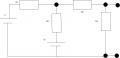# Mesh analysis question help, exam.

Thread Starter

#### accool

Joined Sep 13, 2018
5
hi i got this question on a test and i got it wrong ill show my way of solving it and hopefully someone can tell me what i did wrong and how to solve it.
thanks

Question:and the teacher wanted us to first know the loops and write down the formulas and then write them in standard form.

i used the square on the left as loop 1, and the one on the right as loop 2. i start from the bottom left point of each loop.

loop 1 =v1-R1(i1)-R2(i1-i2)-V2=0

loop 2 =v2-R2(i2)-R3(i1)-R4=0

in my standard form i separated the volts so i used KVL but my friend told me to separate the current so i had to use KCL

and to that im completely oblivious, how do i do that? and are the formulas for my loops even correct?

thank you.

#### bertus

Joined Apr 5, 2008
21,371
Hello,

I moved your thread AGAIN.
Please post this kind of questions in the Homework Help forum and NOT the Digital Design forum.

Bertus

#### KeepItSimpleStupid

Joined Mar 4, 2014
4,787
You can use any convention you want, but I've nearly always used clockwise.

loop 2 =v2-R2(i2)-R3(i1)-R4=0

is in bad form for a number of reasons.

This: loop 2: v2-R2(i2)-R3(i1)-R4=0 is better.

The units would have to be volts or an I * R term.

The -R4 makes the units volts-resistance which isn't right.

Put polarity markings on the battery or use circles with + and - polarites.

Try writing the equations again with clock wise positive and voltage sources with polarity.

You should get in the habit of checking the "dimensions or units" of the answer.

#### WBahn

Joined Mar 31, 2012
26,398
hi i got this question on a test and i got it wrong ill show my way of solving it and hopefully someone can tell me what i did wrong and how to solve it.
thanks

Question:
View attachment 159830

and the teacher wanted us to first know the loops and write down the formulas and then write them in standard form.

i used the square on the left as loop 1, and the one on the right as loop 2. i start from the bottom left point of each loop.

loop 1 =v1-R1(i1)-R2(i1-i2)-V2=0

loop 2 =v2-R2(i2)-R3(i1)-R4=0

in my standard form i separated the volts so i used KVL but my friend told me to separate the current so i had to use KCL

and to that im completely oblivious, how do i do that? and are the formulas for my loops even correct?

thank you.
You should always annotate your drawing with the information you are using in your equations, including both the location and the polarity of any voltages or currents you introduce.

As it is, you don't define the polarity of your two loop currents, leaving us to guess.

Also, if those are marked on your diagram you are less likely to make the mistake you did with R3 in your second equation.

You should always, always, ALWAYS check your units. In your second equation your last term is a resistance but all of the other terms are voltages, so you KNOW it is wrong!

What is meant by "standard form" is going to depend on what you were told standard form is (in other words, there is no standard "standard form").

The most common is to set up a system of linear equations with the terms involving unknowns on the left and terms involving only knowns on the rights, plus with the terms involving unknowns lined up vertically. This makes it easy to put the coefficients in matrix form in order to solve them using classical techniques.

Similar threads